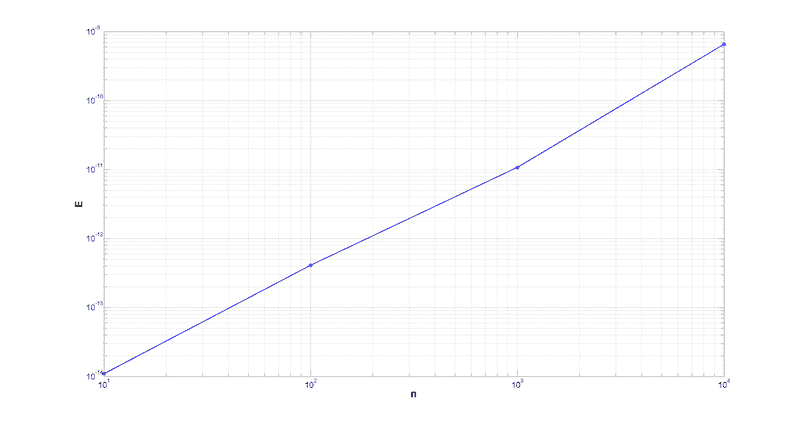# Matlab Extrapolation

Moved from a technical forum, so homework template missing
1. Let A be a random n×n matrix, x = (1,1,...,1)⊤ be an n-vector of ones and b = Ax be the right-hand side vector. As in class, let z = (zj) ∈ Rn be the result of solving the system Ax = b in finite precision using the backslash command. To measure the error between x and z, we let

δ= max |xj−zj|, j =1,...,n

be the maximum componentwise difference between the two vectors. Since A is a random matrix, we need to run this calculation a number of times with different realizations of A in order to get a reasonable value for δ. Let M be the number of trials and suppose that for the kth trial the error is δ(k). We define the mean error as follows:

En = 1/M[δ^(1) +δ^(2) +...+δ^(M)]

Your goal is to determine the approximate size of matrix n = n∗ at which the mean error for Gaussian Elimination is En ≈ 1. In other words, the point at which round-off error in Gaussian elimination is of the same magnitude as the vector x.

In practice, your computer will not have the memory or processing power to find n∗ exactly. Instead, you should extrapolate your data. Find En for reasonable values of n, make a plot of log10(En) versus log10(n) and then perform a suitable extrapolation.

Your conclusions should be explained in a one-page report. Your report should include the following:

(a) A plot of log10(En) versus log10(n).
(b) Justification for the values of n and M you chose.
(c) Explanation of how you do the extrapolation.
(d) An estimation of the number n∗.

Finally, replace A be an random upper-triangular matrix and repeat the process. Hint: you should not need to do the extrapolation step here. You may also wish to plot log10(En) against n here, rather than log10(n).

Code so far:
n = 20:20:500;

M = 200;

mean_error = zeros(length(n),1);

for j = 1:length(n)

error = zeros(M,1);

x = ones(n(j),1);

for i = 1:M

A = randn(n(j),n(j));

b = A*x;

z = A\b;

error(i) = max(abs(z-x));

end

mean_error(j) = mean(error);

mean_error(j)

end

plot(log10(mean_error), log10(n))

Hi everyone, I've attached a .pdf file to show you where I've gotten so far. I'm having a hard time extrapolating the line though, could anyone give me some tips? One of my classes teaching assistants said that polynomial fitting would work well here, but I've tried that and I could not figure it out.

#### Attachments

• extrapolation.pdf
2.2 KB · Views: 265

I've found the curve fitting tool, and I've managed to fit it into a third degree polynomial, but I cannot seem to save the file/call the function correctly to open it in my code...Fantastic. There must be an easier way to do this.

I think it looks like a power function. It is nearly linear on log-log.•JesseJC
I think it looks like a power function. It is nearly linear on log-log.I got it, looks similar to mine. Thanks.